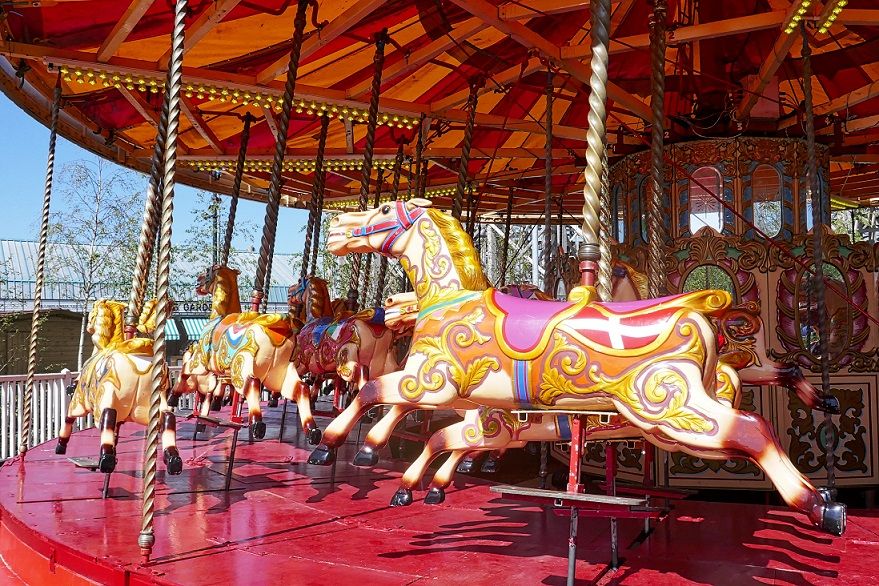# Visualizing Multiclass Classification Results

## Introduction

Visualizing the results of a binary classifier is already a challenge, but having more than two classes aggravates the matter considerably.

Let’s say we have $k$ classes. Then for each observation, there is one correct prediction and $k-1$ possible incorrect prediction. Instead of a $2 \times 2$ confusion matrix, we have a $k^2$ possibilities. Instead of having two kinds of error, false positives and false negatives, we have $k(k-1)$ kinds of errors. And not all errors are created equal: just as we choose an optimal balance of false positives and false negatives depending on the cost associated to each, certain kinds of errors in a multiclass problem will be more or less acceptable. For example, mistaking a lion for a tiger may be acceptable, but mistaking a tiger for a bunny may be fatal.

The goal of visualizing multiclass classification results is to allow the user to quickly and accurately see which errors are occurring and to start developing theories about why those errors are occurring; usually this would be to assist the user during iterative model development, but could also be used, for example, to communicate the behavior of a final classifier to a non-specialized audience.

In this article I employ two basic strategies to try and meet these goals: data visualization techniques and algorithmic techniques. It’s worth a quick reminder about why data visualization is valuable at all. The human visual system is extremely good at picking up certain kinds of patterns (generally those that correspond to spatial relationships and color), but is completely unable to see other kinds of patterns (the digits of $\pi$ coded as greyscale pixel brightness would look like pure noise) and worse yet has a tendency to see patterns in clouds of purely random data where none exist. A good visualization, then, ensures that any interesting structure in the underlying data will be presented in a way that is amenable to interpretation by the human visual system, while any irrelevant or statistically insignificant variation is suppressed.

Algorithmic techniques, on the other hand, do not rely on the human visual system’s ability to detect patterns, but automate the analysis that a human would have done anyway in some procedural way. Rather than merely making it easy to see where a relationship exists, an algorithmic solution would explicitly enumerate and rank the kinds of things the user is interested in. This approach can scale to large data sets much more efficiently, but requires us to trust the algorithm. Both data visualization and algorithmic techniques are useful in practice and are often best when combined.

The underlying problem is very open ended and I do not claim to have come up with any definitive solution, but I did find several novel and useful techniques that seem to me to be worth sharing.

## Case Study

To explore the problem, we need some data and a toy classifier to work with.

The first step is to train and fit some reasonably good but not perfect classifier to some dataset that is reasonably amenable to classification but not linearly separable.

To meet these requirements, I chose the MNIST handwritten digit dataset; This data set is popular for testing multiclass classification algorithms and has the advantage of having very intuitive classes. The MNIST problem is to classify 28x28 gray scale images, which represent center and scaled images of handwritten digits, and assign them to one of ten classes, namely the digits 0 through 9. The MNIST data come pre-labeled and therefore ready to be fed into a supervised learning algorithm. Best of all, it is extremely easy to obtain in an R-friendly format due to its popularity.

As a preprocessing step, we will use T-SNE algorithm provided by the tsne package to reduce the 784 dimensions of the raw pixel data to just two dimensions, which we will simply call x and y.

mnist_tsne <- tsne(as.matrix(mnist_r10000[,1:784]))
xy <- as.data.frame(mnist_tsne)
colnames(xy) <- c('x', 'y')
xy$label <- mnist_r10000$Label


Next, we will apply a multinomial classifier from the nnet package (despite the name, the package actually provides MLP and multinomial log-linear models)

model <- multinom(
label ~ I(x^3) + I(y^3) + I((x^2)*y) + I(x*y^2) + I(x^2) + I(y^2) + x * y,
data=xy,
maxit=500
)